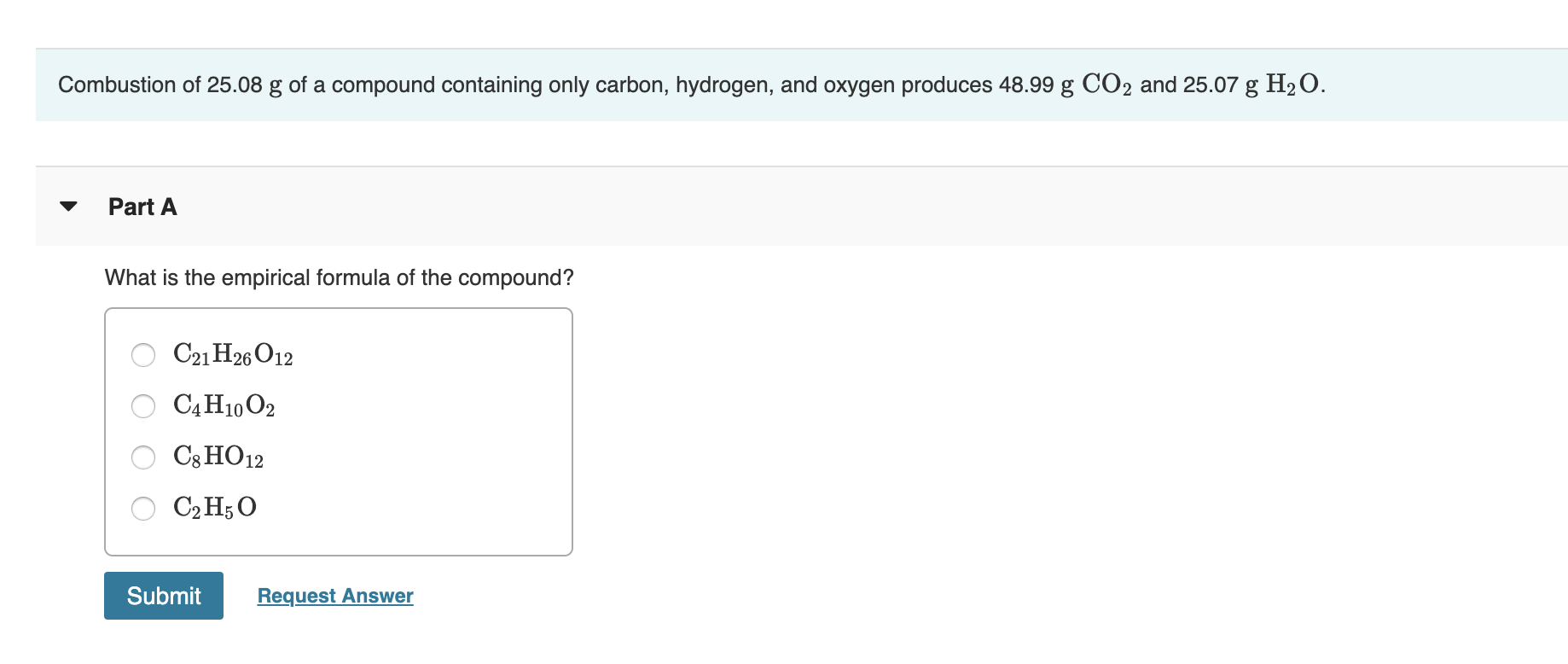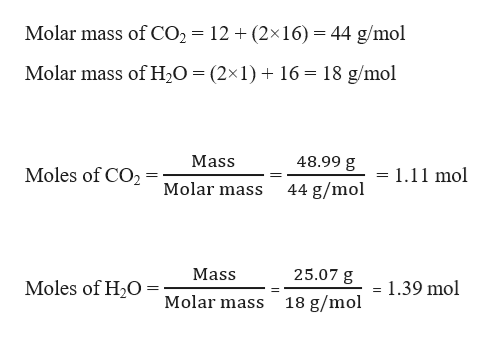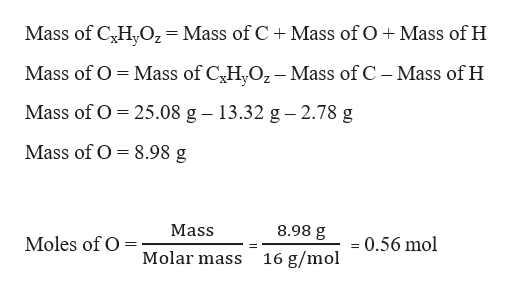# Combustion of 25.08 g of a compound containing only carbon, hydrogen, and oxygen produces 48.99 g CO2 and 25.07 g H2OPart AWhat is the empirical formula of the compound?C21 H26 O12САН10 02C8 HO12СрH5ОSubmitRequest Answer

Question
58 viewshelp_outlineImage TranscriptioncloseCombustion of 25.08 g of a compound containing only carbon, hydrogen, and oxygen produces 48.99 g CO2 and 25.07 g H2O Part A What is the empirical formula of the compound? C21 H26 O12 САН10 02 C8 HO12 СрH5О Submit Request Answer fullscreen
check_circle

Step 1

Let the empirical formula of the compound containing carbon, hydrogen and oxygen be CxHyOz

Given,

Mass of CxHyOz = 25.08 g

Mass of CO2 = 48.99 g

Mass of H2O = 25.07 g

Moles of CO2 and H2O can be calculated as:help_outlineImage TranscriptioncloseMolar mass of CO2 12 (2x16) 44 g/mol Molar mass of H20 = (2x1)16 18 g/mol Mass 48.99 g Moles of CO2 = 1.11 mol Molar mass 44 g/mol 25.07 g Mass Moles of HO=olar mass = 1.39 mol 18 g/mol fullscreen
Step 2

Now, 1 mol of CO2 contains 1 mol of C. Therefore, 1.11 mol of CO2 contains 1.11 mol of C

1 mol of H2O contains 2 mol of H. Therefore, 1.39 mol of H2O contains (2×1.39) 2.78 mol of H.

Mass of C = 1.11 mol × 12 g/mol = 13.32 g

Mass of H = 2.78 mol × 1 g/mol = 2.78 g

Moles of oxygen can be calculated as:help_outlineImage TranscriptioncloseMass of CHO2=Mass of C Mass of O Mass of H Mass of O Mass of CH,O2-Mass of C - Mass of H = Mass of O 25.08 g - 13.32 g 2.78 g Mass of O 8.98 g Mass 8.98 g Moles of O 0.56 mol 16 g/mol Molar mass fullscreen
Step 3

The empirical formula can be computed by f...

### Want to see the full answer?

See Solution

#### Want to see this answer and more?

Solutions are written by subject experts who are available 24/7. Questions are typically answered within 1 hour.*

See Solution
*Response times may vary by subject and question.
Tagged in

### General Chemistry